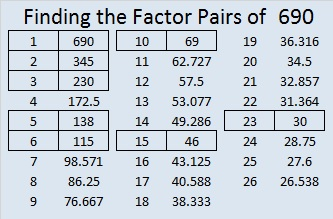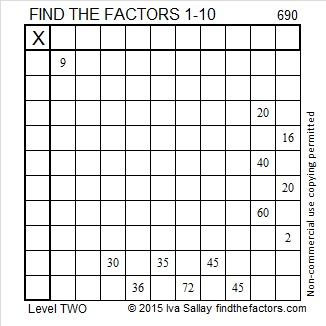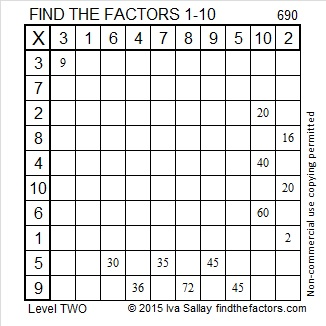# When is 690 a Palindrome? In Base 16 and Base 29.

• 690 is a composite number.
• Prime factorization: 690 = 2 x 3 x 5 x 23
• The exponents in the prime factorization are 1, 1, 1, and 1. Adding one to each and multiplying we get (1 + 1)(1 + 1)(1 + 1)(1 + 1) = 2 x 2 x 2 x 2 = 16. Therefore 690 has exactly 16 factors.
• Factors of 690: 1, 2, 3, 5, 6, 10, 15, 23, 30, 46, 69, 115, 138, 230, 345, 690
• Factor pairs: 690 = 1 x 690, 2 x 345, 3 x 230, 5 x 138, 6 x 115, 10 x 69, 15 x 46, or 23 x 30
• 690 has no square factors that allow its square root to be simplified. √690 ≈ 26.267851Here is today’s factoring puzzle:Print the puzzles or type the solution on this excel file: 10 Factors 2015-11-23

———————————————————————————

Here is a little more about the number 690:

690 is the sum of the six prime numbers from 103 to 131. Do you know what all of those prime numbers are?

690 is also the hypotenuse of Pythagorean triple 414-552-690. What is the greatest common factor of those three numbers?

In BASE 10 we use the digits 0, 1, 2, 3, 4, 5, 6, 7, 8, and 9. For bases smaller than 10 we use only the digits less than that base. For bases greater than 10, we might use A to represent 10, B to represent 11, and so forth all the way to Z representing 35 in BASE 36.

690 is a palindrome in two bases that require us to use letters of the alphabet to represent it:

• 2B2 in BASE 16; note that 2(256) + 11(16) + 2(1) = 690
• NN in BASE 29; note that 23(29) + 23(1) = 690. (N is the 14th letter of the alphabet and 14 + 9 = 23)

NN looks like it is divisible by 11, but remember that 11 base 29 is the same as 30 in base 10.

———————————————————————————This site uses Akismet to reduce spam. Learn how your comment data is processed.